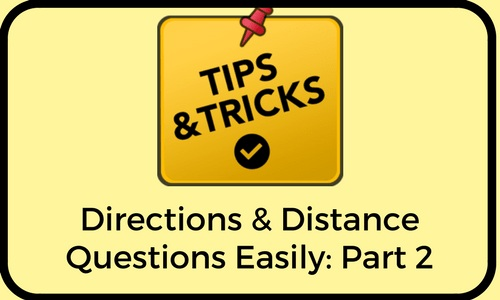# Tricks to Solve Directions Sense Ques: Part 2In this article we learn how to solve directions and distances easily by developing tricks

#### TRICK 1:

If only one person covers the distance

• E+E+E+E+E+....
• W+W+W+W+.....
• N+N+N+N+....
• S+S+S+S+......

#### TRICK 2:

In opposite direction subtract
• E-W
• W-E
• N-S
• S-N

### QUESTION 1:

Arun moves towards East and covers a distance of 10m then turns North and covers a distance of 20m turns East of 20m and turns North of 20m. How far in which direction is he from his initial position?

#### TRICK:

=E10 N20 E20 N20 (E10+E20 N20+N20)
=E30 N40
=square root of 900+1600 = NE50m

### QUESTION 2:

Raju Facing south turns clockwise with an angle of 45 degrees turns anti clockwise angle of 90degrees then turns clockwise of 135degrees then turns anti-clockwise angle of 90degrees then turns clockwise of 45degrees. In which direction is he facing now?

#### TRICK:

=C45 A90 C135 A90 C45 (C45+C135+C45 A90+A90)
=C225 A180
=C45=45degrees from south is SW

### QUESTION 3:

Raju moves towards East and covers a distance of 20m then turns left and covers a distance of 30m turns right of 40m, turns left of 50m, turns right of 45degrees. In which direction is he facing?

#### TRICK:

=E20 N30 E40 N50 E45degrees

### Question 4:

Raju facing towards NE turns clockwise of 20degrees , turns anti-clockwise of 35degrees, turns clockwise of 30degrees, turns anti-clockwise of 55degrees, turns clockwise of 40degrees. In which direction is he facing?

#### TRICK:

Without pen on paper
=C20 A35 C30 A55 C40
=C90 A90 (C90-A90=0)
=0=NE (Initial position)